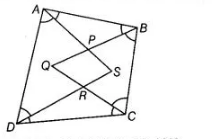# Prove the following`
Question:

If bisectors of $\angle A$ and $\angle B$ of a quadrilateral $A B C D$ intersect each other at $P$, of $\angle B$ and $\angle C$ at $Q$, of $\angle C$ and $\angle D$ at $R$ and of $\angle D$ and $\angle A$ at $S$, then $P Q R S$ is a

(a) rectangle

(b) rhombus

(c) parallelogram

(d) quadrilateral whose opposite angles are supplementary

Solution:

(d) Given, $A B C D$ is a quadrilateral and all angles bisectors form a quadrilateral $P Q R S$.We know that, sum of all angles in a quadrilateral is $360^{\circ}$.

$\therefore \quad \angle A+\angle B+\angle C+\angle D=360^{\circ}$

On dividing both sides by 2 , we get

$\frac{1}{2}(\angle A+\angle B+\angle C+\angle D)=\frac{360^{\circ}}{2}$

$\Rightarrow \quad \angle P A B+\angle P B A+\angle R C D+\angle R D C=180^{\circ}$ $\ldots(i)$

[since, $A P$ and $P B$ are the bisectors of $\angle A$ and $\angle B$ respectively also $R C$ and $R D$ are the bisectors of $\angle C$ and $\angle D$ respectively]

Now, in $\triangle A P B$,

$\angle P A B+\angle A B P+\angle B P A=180^{\circ}$

[by angle sum property of a triangle]

$\Rightarrow \quad \angle P A B+\angle A B P=180^{\circ}-\angle B P A \quad$... (ii)

Similarly in $\triangle R D C$,

$\angle R D C+\angle D C R+\angle C R D=180^{\circ}$

[by angle sum property of a triangle]

$\Rightarrow \quad \angle R D C+\angle D C R=180^{\circ}-\angle C R D \quad \ldots$ (ii)

On substituting the value Egs. (ii) and (iii) in Eg. (i), we get

$180^{\circ}-\angle B P A+180^{\circ}-\angle D R C=180^{\circ}$

$\Rightarrow \quad \angle B P A+\angle D R C=180^{\circ}$

$\Rightarrow \quad \angle S P Q+\angle S R Q=180^{\circ}$

$[\because \angle B P A=\angle S P Q$ and $\angle D R C=\angle S R Q$ vertically opposite angles $]$

Hence, $P Q R S$ is a quadrilateral whose opposite angles are supplementary.